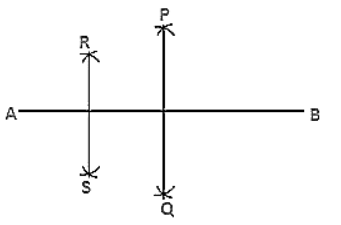# Draw a line segment AB bisect it.

Question:

Draw a line segment AB bisect it. Bisect one of the equal parts to obtain a line segment of length (1/4) (AB).

Solution:

Steps of construction:

1. Draw a line segment AB.

2. With A as center and radius more than half of AB draw arcs, one on each side of AB.

3. With B as the center and same radius draw arcs cutting the previous arcs at points P and Q respectively.

4. Join P and Q which intersects AB at C.

5. With A as center and radius more than half of AC draw arcs, one on each side of AC.

6. With C as the center and same radius draw arcs cutting the previous arcs at R and S respectively.

7. Join points R and S which intersects AC at D.